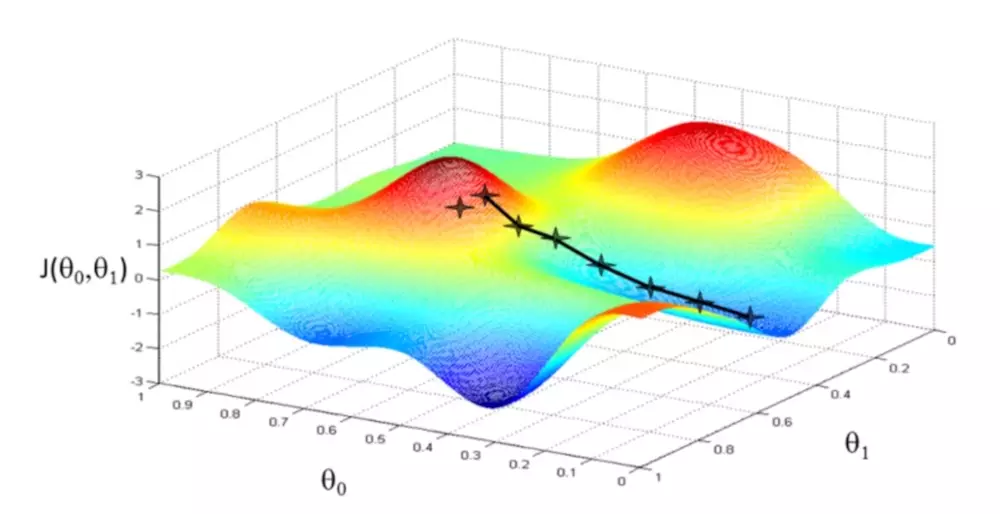# 理解线性回归算法

## 基本概念

• 代价函数/损失函数 (Cost fuction)

:线性回归的目标是让 假设函数与训练集尽量的拟合，使得代价函数最小,前面的 $1/2$的作用是因为平方误差函数求导之后正好抵消,使得在梯度下降里面的偏导数项系数正好为1

$$J(\theta\_0, \theta\_1) = \frac{1}{2m} \sum\_{i=1}^{m}(h\_\theta(x^{(i)}) - y^{(i)})^2$$

## 梯度下降算法

### 梯度下降公式$$\theta_j := \theta_j - \alpha\frac{\partial}{\partial\theta_j}J(\theta_0,\theta_1)$$

### 理解梯度

• 梯度即变化率最大的那个方向导数
• 偏导数是一类特殊的方向导数
• 求得某点的各方向偏导数, 可以构成一个向量, 沿着该向量变化最大, 即方向导数

## 多元线性回归

begin{align}
hat{y} = omega_{0} + sum_{i=1}^{k}omega_{i}x_{i}
end{align}

begin{align}
loss^{R} = frac{1}{2m}sum_{i=1}^{m}(hat{y}^{(i)} - y^{(i)})^2
end{align}

begin{align}
frac{partial{loss^{R}}}{partialtheta} = begin{cases}
frac{1}{m}sum_{i=1}^{m}(hat{y}^{(i)} - y^{(i)}) & text{if } theta text{ equals to } omega_{0} \\
\\
\\
frac{1}{m}x_{t}sum_{i=1}^{m}(hat{y}^{(i)} - y^{(i)}) & text{if } theta text{ equals to } omega_{t}
end{cases}
end{align}

• Batch Gradient Descent (BGD) 批量梯度下降, 每次迭代使用全部训练数据
• Stochastic Gradient Descent (SGD) 随机梯度下降, 每次仅仅选取一个样本来求梯度
• Mini-Batch Gradient Descent (MBGD) 小批量梯度下降，每次抽取x个样本进行训练

## 代码实现

https://github.com/Tara-X/algo/tree/master/linear